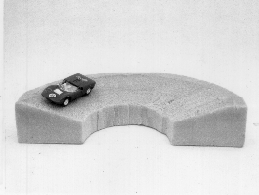D1-51.HTMLD1-51: BANKED CURVE MODEL

PURPOSE: To aid in explaining why curved roads are banked.

DESCRIPTION: The model of the curved road is banked such that at the suggested maximum rate of speed the horizontal component of the normal force provides the centripetal force required to keep the car moving in its circular path, independent of the friction of the car wheels with the road. Referring to the diagram below, where N is the normal force, m is the mass of the car, v is the suggested maximum speed, r is the radius of the curve, g is the acceleration of gravity, and a is the banking angle, then N cos a = mg and N sin a = mv^2/r, so tan a = v^2/gr.SUGGESTIONS: A ball can be used to illustrate this effect as follows: roll the ball along the path of the car very slowly and it will roll off the inner edge; roll the ball very rapidly and it will leave the upper edge; roll the ball with the suggested speed and it will roll along the center of the banked curve, as in the case of a car with no friction between the wheels and the road.

REFERENCES: (PIRA 1D50.60)

EQUIPMENT: Banked styrofoam curve model with toy car and ball.

SETUP TIME: None.

Go back to Lecture-Demonstration Home Page.